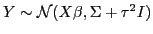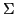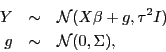Next: Standard errors in gam(), Up: Spatial and spatio-temporal data Previous: Space-time smoothing in R

#### Universal kriging and generalized least squares (GLS) fitting

If you're interested in fitting a model,, wherecaptures correlation, such as spatial structure, you have two options. I prefer likfit() for two reasons. First it can make use of the Matérn covariance. Second it seems to do a much better maximization job, often finding higher likelihoods than gls().

1. Use gls() from the nlme package in R. The 'exponential' and 'Gaussian' correlation functions are two options. Make sure to include the nugget. Note that 'sig2' is the total variation and 'nugget' is the proportion for the spatial component (I think - I haven't checked this recently).
gls(y~x,correlation=corExp(form=~xs1+xs2,nugget=TRUE),method='ML')
2. Use likfit() from the geoR package in R. Here trend.spatial specifies the mean term. kappa is the Matérn differentiability parameter.
likfit(coords=xs,data=y,ini.cov.pars=c(0.4,0.4),fix.kappa=T,kappa=2,cov.model='matern',trend=trend.spatial(~x),messages=F,method.lik='ML')
Note that the model above is the same as a modelnamely a spatial random effects or Gaussian process-based model.

Last modified: 12/14/08. Typo corrected 1/14/12.

Chris Paciorek 2012-01-21